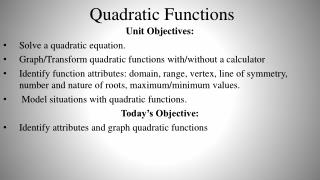DownloadDownload PresentationTélécharger la présentation- - - - - - - - - - - - - - - - - - - - - - - - - - - E N D - - - - - - - - - - - - - - - - - - - - - - - - - - -
##### Presentation Transcript

1. Quadratic Functions Unit Objectives: Solve a quadratic equation. Graph/Transform quadratic functions with/without a calculator Identify function attributes: domain, range, vertex, line of symmetry, number and nature of roots, maximum/minimum values. Model situations with quadratic functions. Today’s Objective: Identify attributes and graph quadratic functions

2. Quadratic Function: where • Graph: • Parabola • Parent function/equation: • Vertex • Point where graph changes direction • Minimum or maximum • Vertex Form: • Vertex: (h, k) • Axis of Symmetry (line) • Divides the graph into 2 mirror images • x = h

3. Transformation of Translation: Vertical Translation: Horizontal Right h units Up k units Down k units Left h units Reflection Dilation: Across x-axis Stretch: Vertex Form: Compression:

4. Graphing a Quadratic Function in vertex form • Vertex: Plot the vertex Find and plot two points to the right of vertex. Plot the pointacross axis of symmetry. Sketch the curve. Axis of Symmetry: Domain: Range: All Real Numbers

5. Graphing a Quadratic Function in vertex form • Vertex: Plot the vertex Find and plot two points to the right of vertex. Plot the pointacross axis of symmetry. Sketch the curve. Axis of Symmetry: Domain: Range: All Real Numbers

6. Graphing a Quadratic Function in vertex form • Vertex: Plot the vertex Find and plot two points to the right of vertex. Plot the pointacross axis of symmetry. Sketch the curve. Axis of Symmetry: Domain: Range: All Real Numbers

7. Writing a Quadratic function: vertex form • Identify the Vertex: • Finding dilation factor: • Choose another known point and solve for a. (-1, -5) (-2, -7)

8. Writing a Quadratic function: vertex form (3, 9) • Identify the Vertex: (5, 7) • Finding dilation factor: • Choose another known point and solve for a. Pg. 199#7-37 odds